×
Get Full Access to Introductory Chemistry - 5 Edition - Chapter 11 - Problem 34p
Get Full Access to Introductory Chemistry - 5 Edition - Chapter 11 - Problem 34p

×

Solved: A sample of gas has an initial volume of 22.8 L at a pressure of 1.65 atm. IfISBN: 9780321910295 34

Solution for problem 34P Chapter 11

Introductory Chemistry | 5th Edition

• Textbook Solutions
• 2901 Step-by-step solutions solved by professors and subject experts
• Get 24/7 help from StudySoup virtual teaching assistantsIntroductory Chemistry | 5th Edition

4 5 1 278 Reviews
29
5
Problem 34P

PROBLEM 34P

A sample of gas has an initial volume of 22.8 L at a pressure of 1.65 atm. If the sample is compressed to a volume of 10.7 L, what is its pressure? (Assume constant temperature.)

Step-by-Step Solution:

PROBLEM 34P

A sample of gas has an initial volume of 22.8 L at a pressure of 1/65 atm. If the sample is compressed to a volume of 10.7 L, what is it’s pressure? (Assume constant temperature)

Step by Step Solution

Step 1 of 2

According to Boyle’s law, volume of a given amount of gas at constant temperature is inversely proportional to the pressure of gas.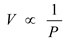PV = constant

For two different set of conditions,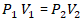Where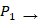initial pressure of the gas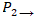Final pressure of the gas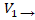Initial volume of the gas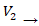Final pressure of the gas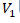Step 2 of 2

ISBN: 9780321910295

Since the solution to 34P from 11 chapter was answered, more than 1810 students have viewed the full step-by-step answer. This full solution covers the following key subjects: Volume, sample, pressure, initial, gas. This expansive textbook survival guide covers 19 chapters, and 2046 solutions. Introductory Chemistry was written by and is associated to the ISBN: 9780321910295. The answer to “A sample of gas has an initial volume of 22.8 L at a pressure of 1.65 atm. If the sample is compressed to a volume of 10.7 L, what is its pressure? (Assume constant temperature.)” is broken down into a number of easy to follow steps, and 35 words. The full step-by-step solution to problem: 34P from chapter: 11 was answered by , our top Chemistry solution expert on 05/06/17, 06:45PM. This textbook survival guide was created for the textbook: Introductory Chemistry, edition: 5.

Unlock Textbook Solution